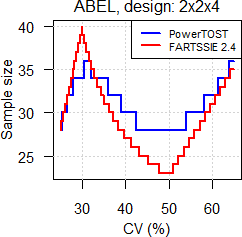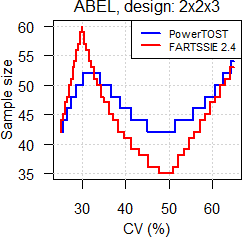## Run on R v4.0.3 [Power / Sample Size]

Hi Yung-jin,

❝ ❝ Sample size: 18

❝ ❝ Runtime (seconds):

❝ ❝ user system elapsed

❝ ❝ 0.14 0.01 0.15

❝ I run the codes three times under R v4.0.3 and got:

Runtime (seconds):

   user  system elapsed

   0.11    0.00    0.13

   0.09    0.01    0.10

   0.11    0.00    0.11

❝ a little bit faster.I guess that you have not upgraded to R 4.0.3 yet.

Nope, was on 4.0.3 as well.

❝ R v4.0.3 is simply faster (still my gut feeling).

Cannot check. Deleted 4.0.2 already and not in the mood to reinstall it.

❝ I remember that your Xeon computer runs faster than my Windows, even than my Linux notebook last time. Is it the same computer?

It is. However, median of 15 runs 0.16 seconds. No idea.

❝ ❝ BTW, in v2.5 of last Tuesday Dave removed all reference-scaled methods and gives the code of PowerTOST in a box.❝ Thanks for the information.

Welcome.

Not for an initiate like you but for FARTSSIE-adepts…
With the script below you can reproduce the results of FARTSSIE ≤2.4; for the EMA’s ABEL you have to change cell U16 from 5 (which is for Health Canada’s TPD) to 3.

library(PowerTOST) CV     <- seq(0.25, 0.65, 0.05) theta0 <- 0.90 power  <- 0.80 design <- "2x2x4" pers   <- as.numeric(substr(design, 5, 5)) n      <- data.frame(CV = CV) theta1 <- numeric() for (j in seq_along(CV)) {   theta1[j] <- exp(-0.76*CV2se(CV[j]))   if (CV[j] <= 0.3) theta1[j] <- 0.8   if (CV[j] >= 0.5) theta1[j] <- exp(-0.76*CV2se(0.5))   n.2x2x2       <- sampleN.TOST(CV = CV[j], design = "2x2x2",                                 targetpower = power, theta0 = theta0,                                 theta1 = theta1[j], theta2 = 1/theta1[j],                                 method = "nct", details = FALSE,                                 print = FALSE)[["Sample size"]]   n$FARTSSIE[j] <- ceiling(n.2x2x2*(-0.25*pers+1.5)) n$ABEL[j]     <- sampleN.scABEL(CV = CV[j], design = design,                                   targetpower = power, theta0 = theta0,                                   details = FALSE,                                   print = FALSE)[["Sample size"]] } n$CV <- n$CV*100 names(n) <- "CV (%)" cat("design:", design, "\n"); print(n, row.names = FALSE)design: 2x2x4  CV (%) FARTSSIE ABEL      25       28   28      30       40   34      35       32   34      40       27   30      45       25   28      50       23   28      55       27   30      60       31   32      65       35   36design: 2x2x3  CV (%) FARTSSIE ABEL      25       42   42      30       60   50      35       48   50      40       41   46      45       38   42      50       35   42      55       41   44      60       47   48      65       53   54

Flaws:
• Direct expansion of the limits instead of simulations, restriction of the point estimate (within 80.00–125.00%) not taken into account.
• Sample size estimated for 2×2×2 design and adapted (i.e., 50% for 4-period full replicates, 75% for three period replicates).
PS: Do you plan to implement my-code for ABE of last May in a future release of bear?

Dif-tor heh smusma 🖖🏼 Довге життя Україна!Helmut SchützThe quality of responses received is directly proportional to the quality of the question asked. 🚮
Science QuotesIng. Helmut Schütz